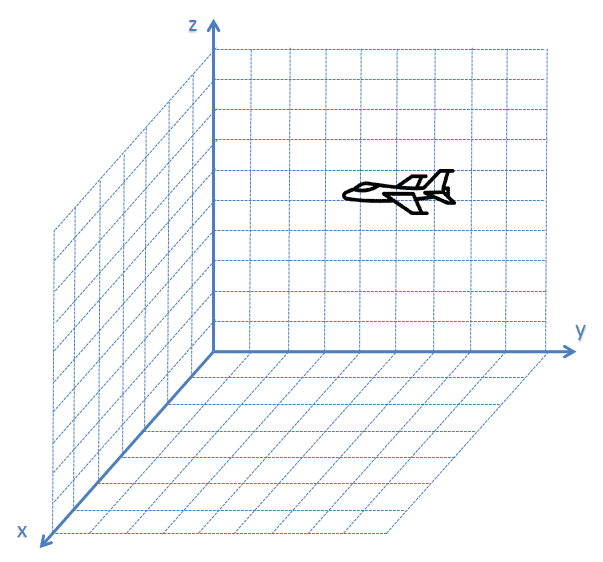﻿ Multivariate Kalman Filter

# Multivariate Kalman Filter

After reading the "Kalman Filter in one dimension" section, you should be familiar with the concepts of the Kalman Filter. In this section, we derive the multidimensional (multivariate) Kalman Filter equations.

This tutorial section deals with a Linear Kalman Filter (LKF). The LKF assumes that the system dynamics are linear.

Until now, we've dealt with one dimensional processes, like estimating the liquid temperature. But many dynamic processes have two, three, or even more dimensions.

For instance, the state vector that describes the airplane's position in space is three-dimensional:

$\left[ \begin{matrix} x\\ y\\ z\\ \end{matrix} \right]$

The state vector that describes the airplane position and velocity is six-dimensional:

$\left[ \begin{matrix} x\\ y\\ z\\ \dot{x}\\ \dot{y}\\ \dot{z}\\ \end{matrix} \right]$

The state vector that describes the airplane position, velocity, and acceleration is nine-dimensional:

$\left[ \begin{matrix} x\\ y\\ z\\ \dot{x}\\ \dot{y}\\ \dot{z}\\ \ddot{x}\\ \ddot{y}\\ \ddot{z}\\ \end{matrix} \right]$Assuming a constant acceleration dynamic model, we can describe the extrapolated airplane state at time $$n$$ by nine motion equations:

$\begin{cases} x_{n} = x_{n-1} + \dot{x}_{n-1} \Delta t+ \frac{1}{2}\ddot{x}_{n-1} \Delta t^{2}\\ y_{n} = y_{n-1} + \dot{y}_{n-1} \Delta t+ \frac{1}{2}\ddot{y}_{n-1} \Delta t^{2}\\ z_{n} = z_{n-1} + \dot{z}_{n-1} \Delta t+ \frac{1}{2}\ddot{z}_{n-1} \Delta t^{2}\\ \dot{x}_{n} = \dot{x}_{n-1} + \ddot{x}_{n-1} \Delta t\\ \dot{y}_{n} = \dot{y}_{n-1} + \ddot{y}_{n-1} \Delta t\\ \dot{z}_{n} = \dot{z}_{n-1} + \ddot{z}_{n-1} \Delta t\\ \ddot{x}_{n} = \ddot{x}_{n-1}\\ \ddot{y}_{n} = \ddot{y}_{n-1}\\ \ddot{z}_{n} = \ddot{z}_{n-1}\\ \end{cases}$

It is common practice to describe a multidimensional process with a single equation in matrix form.

First, it is very exhausting to write all these equations; representing them in matrix notation is much shorter and more elegant.

Second, computers are highly efficient at matrix calculations. Implementing the Kalman Filter in matrix form yields faster computation run time.

The following chapters describe the Kalman Filter equations in matrix form. And, of course, the theoretical part is followed by fully solved numerical examples.

The final chapter includes two numerical examples. In the first example, we design a six-dimensional Kalman Filter without control input. In the second example, we design a two-dimensional Kalman Filter with a control input.